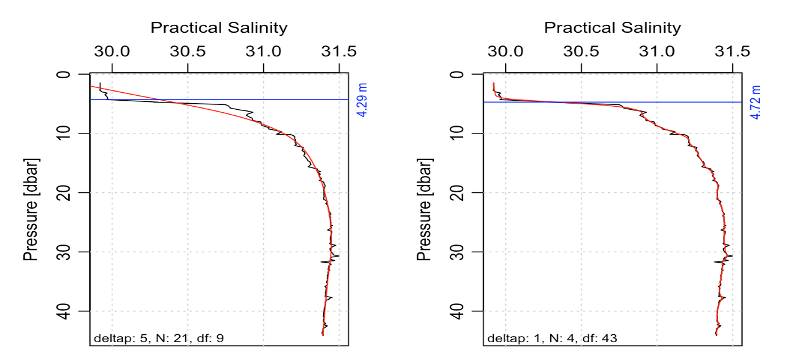# Introduction

There are no agreed-upon methods for inferring halocline depth, but a reasonable method might involve locating the depth at which dS/dp is largest, where S is salinity and p is pressure (Kelley 2014 chapter 5). Calculating the derivative using e.g. `diff(S)/diff(p)` can be problematic because of sensitivity to noise, especially for data that have not been bin-averaged. Achieving smoothness with conventional filtering has problems at the end-points, which is particularly troublesome for a near-surface halocline (see the next blog entry). A possible solution to such problems is to calculate the derivative with a smoothing spline.

# Methods

Pasted below is test code that does this with the ctd dataset in the oce package. The function returns the pressure at which the smoothing spline has highest salinity derivative, and it can also plot the results (which is recommended). The parameter named deltap is used to set the value of `df` (degrees of freedom) for the spline. One might think of deltap as the thickness (in dbar) of the smoothing interval for each of the sub-components of the spline.

# Results

The graph shows results for a test dataset provided with the oce package, using two values for `deltap`.# Discussion

Lacking further information about the data or a statistical model of the variation, the choice of deltap is somewhat open, and one interpretation of the results would be to state that the halocline depth is likely to be between 4 and 5 m.

This website is written in Jekyll, and the source is available on GitHub.